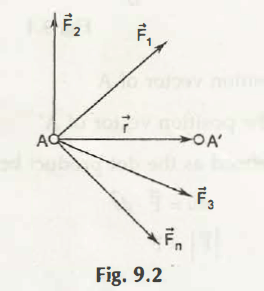Let us consider a particle A displaces to A’ due to several external forces F1, F2, F3….. Fn. This imagined displacement to become A’ is virtual displacement as shown in Fig. 9.2Then, virtual work can be given by-

u = F1.r + F2.r + F3.r +……+ Fn.r

= (F1+ F2 + F3 +……+ Fn)r

= R.r

Where,

R = Resultant of all the forces

Or,

It can be defined as sum of virtual work of every single force is equal to its resultant.

Suppose the body is in equilibrium while applying the force. In this condition, the resultant R will be equal to 0 (zero).

The above statement helps in defining the principle of virtual work. It states that total virtual work of forces will be zero for any displacement when the body is in equilibrium.

Links of Next Mechanical Engineering Topics:-### Customer Reviews

My Homework Help
Rated 5.0 out of 5 based on 510 customer reviews at